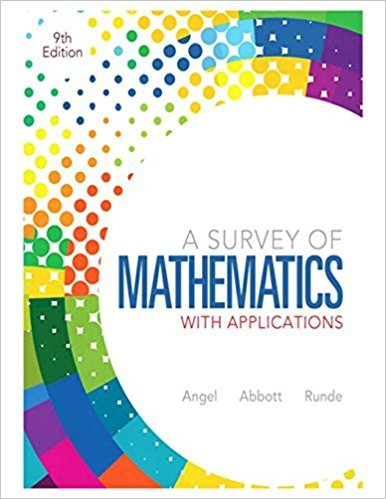×
×

# Solutions for Chapter 6.4: Algebra, Graphs, and Functions## Full solutions for A Survey of Mathematics with Applications | 9th Edition

ISBN: 9780321759665Solutions for Chapter 6.4: Algebra, Graphs, and Functions

Solutions for Chapter 6.4
4 5 0 330 Reviews
20
4
##### ISBN: 9780321759665

Since 53 problems in chapter 6.4: Algebra, Graphs, and Functions have been answered, more than 74330 students have viewed full step-by-step solutions from this chapter. A Survey of Mathematics with Applications was written by and is associated to the ISBN: 9780321759665. Chapter 6.4: Algebra, Graphs, and Functions includes 53 full step-by-step solutions. This expansive textbook survival guide covers the following chapters and their solutions. This textbook survival guide was created for the textbook: A Survey of Mathematics with Applications, edition: 9.

Key Math Terms and definitions covered in this textbook
• Condition number

cond(A) = c(A) = IIAIlIIA-III = amaxlamin. In Ax = b, the relative change Ilox III Ilx II is less than cond(A) times the relative change Ilob III lib II· Condition numbers measure the sensitivity of the output to change in the input.

• Eigenvalue A and eigenvector x.

Ax = AX with x#-O so det(A - AI) = o.

• Elimination.

A sequence of row operations that reduces A to an upper triangular U or to the reduced form R = rref(A). Then A = LU with multipliers eO in L, or P A = L U with row exchanges in P, or E A = R with an invertible E.

• Four Fundamental Subspaces C (A), N (A), C (AT), N (AT).

Use AT for complex A.

• Free variable Xi.

Column i has no pivot in elimination. We can give the n - r free variables any values, then Ax = b determines the r pivot variables (if solvable!).

• Graph G.

Set of n nodes connected pairwise by m edges. A complete graph has all n(n - 1)/2 edges between nodes. A tree has only n - 1 edges and no closed loops.

• Hermitian matrix A H = AT = A.

Complex analog a j i = aU of a symmetric matrix.

• Hilbert matrix hilb(n).

Entries HU = 1/(i + j -1) = Jd X i- 1 xj-1dx. Positive definite but extremely small Amin and large condition number: H is ill-conditioned.

• Identity matrix I (or In).

Diagonal entries = 1, off-diagonal entries = 0.

• Left nullspace N (AT).

Nullspace of AT = "left nullspace" of A because y T A = OT.

• Linear combination cv + d w or L C jV j.

• Normal equation AT Ax = ATb.

Gives the least squares solution to Ax = b if A has full rank n (independent columns). The equation says that (columns of A)·(b - Ax) = o.

• Particular solution x p.

Any solution to Ax = b; often x p has free variables = o.

• Positive definite matrix A.

Symmetric matrix with positive eigenvalues and positive pivots. Definition: x T Ax > 0 unless x = O. Then A = LDLT with diag(D» O.

• Rank r (A)

= number of pivots = dimension of column space = dimension of row space.

• Row space C (AT) = all combinations of rows of A.

Column vectors by convention.

• Schwarz inequality

Iv·wl < IIvll IIwll.Then IvTAwl2 < (vT Av)(wT Aw) for pos def A.

• Spectrum of A = the set of eigenvalues {A I, ... , An}.

Spectral radius = max of IAi I.

• Transpose matrix AT.

Entries AL = Ajj. AT is n by In, AT A is square, symmetric, positive semidefinite. The transposes of AB and A-I are BT AT and (AT)-I.

• Triangle inequality II u + v II < II u II + II v II.

For matrix norms II A + B II < II A II + II B II·

×